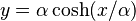# Catenary

## Definition

### Cartesian and parametric equations

The Cartesian equation for the catenary is:$y = \alpha \cosh (x/\alpha)$

where$\alpha$ is a parameter determining how much the catenary opens up.

### Physical definition

The catenary is described as follows: it is the shape taken by a hanging flexible wire under the influence of uniform gravitational force, when supported at the ends. Here, the downward direction gravitationally corresponds to the negative$y$-axis, and the parameter$\alpha$ is related to the extent of gravitational force.

## Constructs

A surface of revolution corresponding to this planar curve is: catenoid